The International Information Center for Structural Engineers

Thursday, 30 July 2015 09:45cat

## Calculation Example: Natural Periods of Vibration for Systems

Written by  TheStructuralEngineer.info

Each of the following columns supports a block of identical mass, m. The columns are fixed at the bottom and free at the top. The height of the first column is twice the height of the second column. The modulus of elasticity, E, and moment of inertia, I, for both columns are the same. The systems have natural periods of vibration of T1 and T2, respectively. Neglecting the weight of the columns, what is the natural period of vibration for the first system?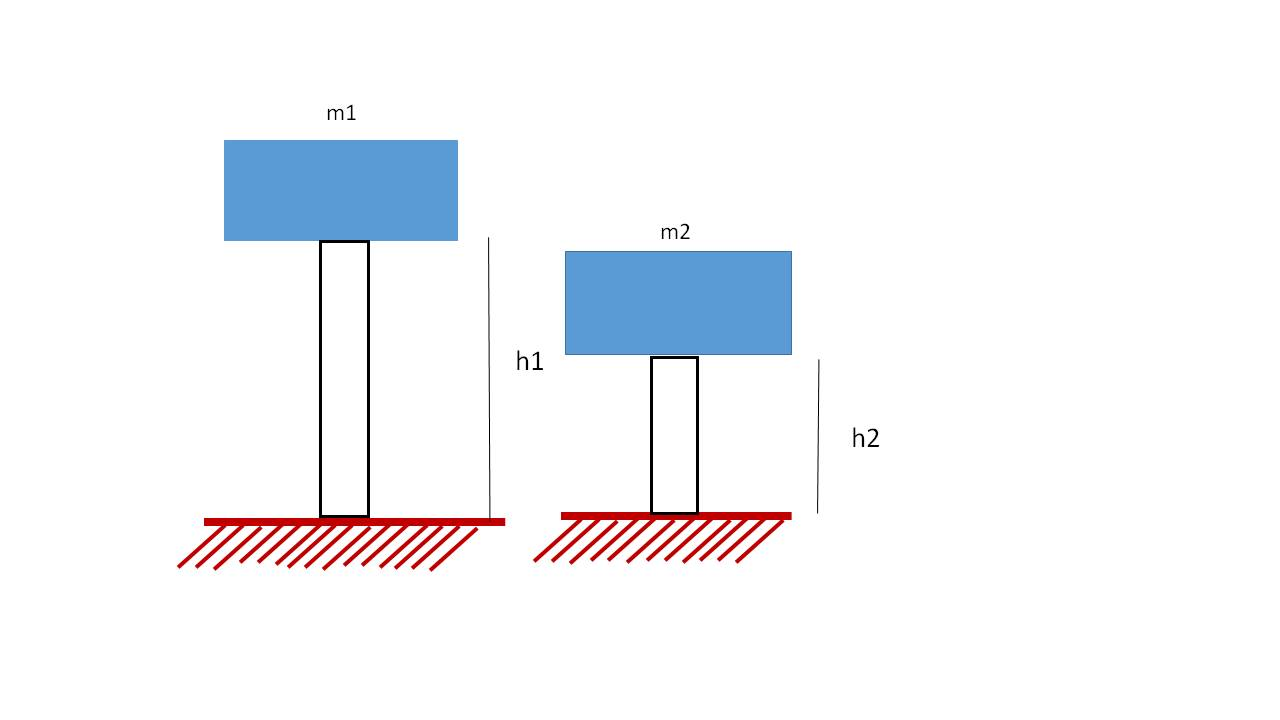a. 0.2T2

b. 0.2T2

c. 2T2

d. 3T2

SI solution

The stiffness of a cantilever beam is described by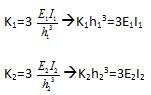It is known that E1=E2 and I1=I2. Since h1=2h2, then: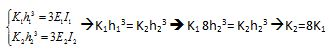Since K2=8K1 , then:

System 1: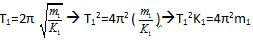System 2: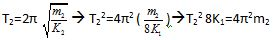Given that m1=m2, it is assumed: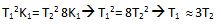• Default
• Title
• Date
• Random
• ## Keller lays foundation for Aston Martin tower

Keller business units HJ Foundation and Hayward Baker worked on the foundation system of one of Miami's most prestigious, eye-catching